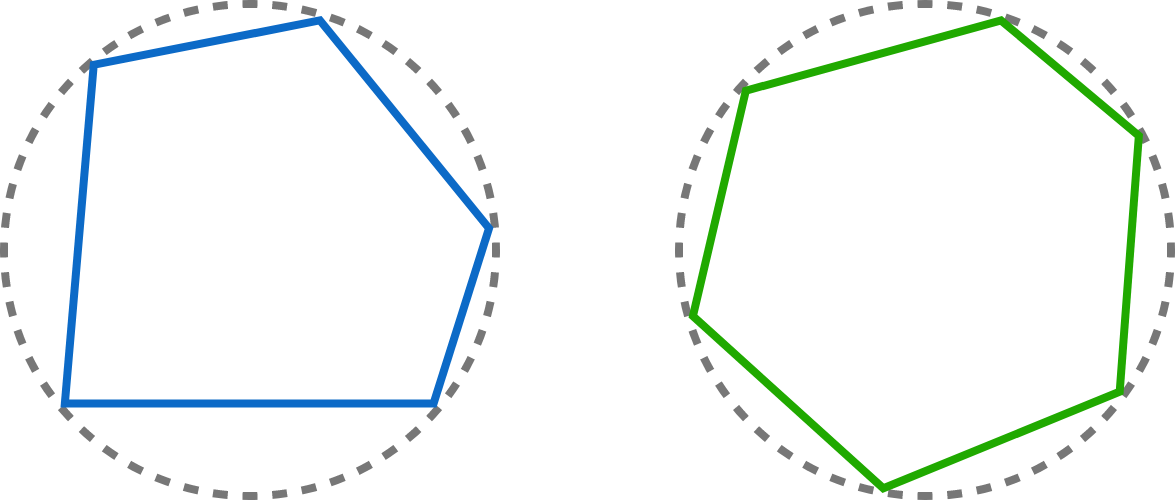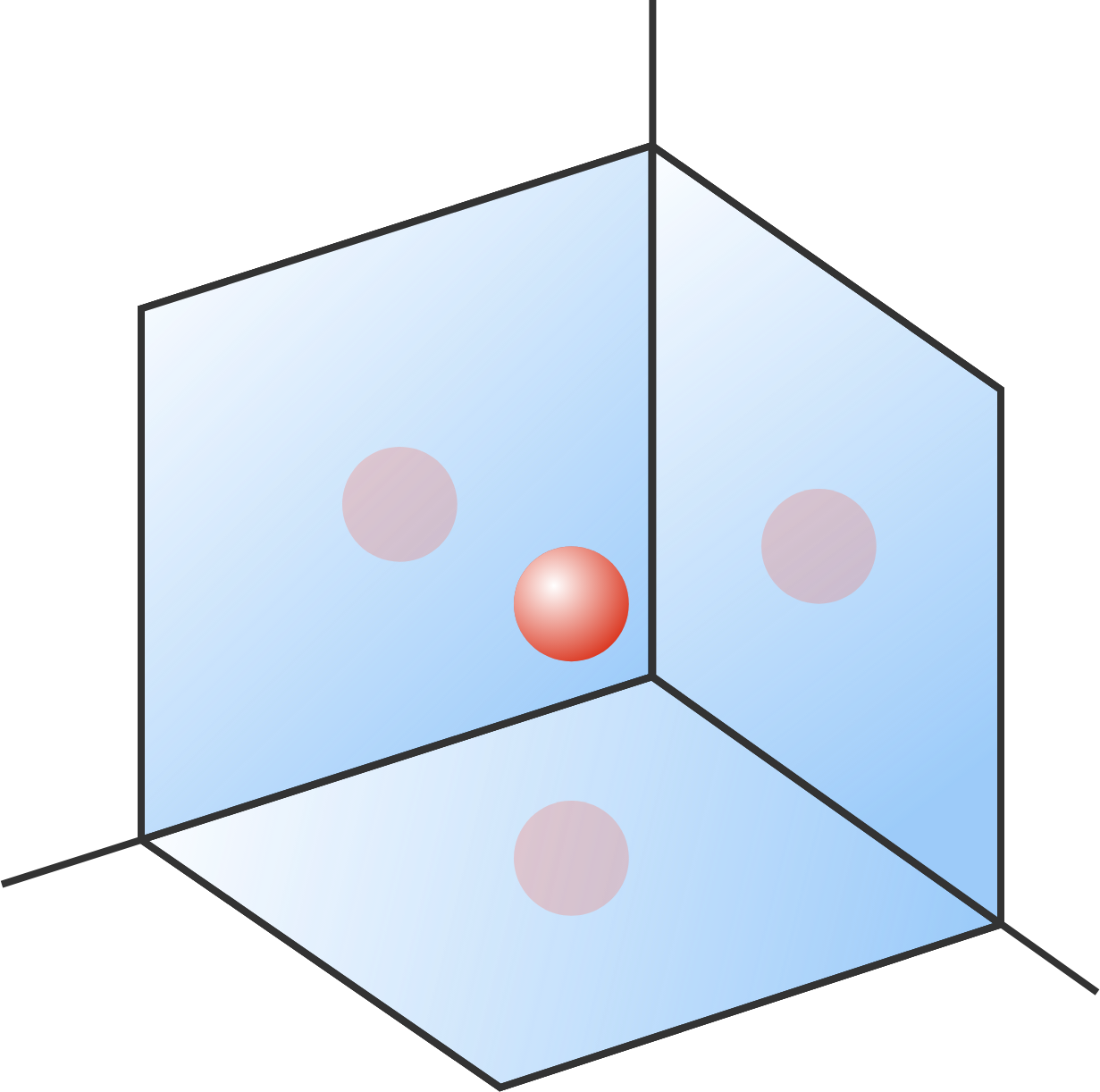# Problems of the Week

Contribute a problem

# 2017-07-31 Intermediate

$\large{\begin{array}{ccc} && & {\color{#3D99F6}E} & {\color{#20A900}O} \\ && & & {\color{#EC7300}O}\\ + && & & {\color{#69047E}O}\\ \hline & & & E & {\color{#D61F06}O}\\ \end{array}}$

The $E$ positions in the cryptogram above indicate even digits and the $O$ positions indicate odd digits. No digit is repeated. While there is not a unique way to fill in the cryptogram, the red $\color{#D61F06}O$ in the sum's result can only be one particular value. What is it?

True or False?

1. A cyclic $\color{#3D99F6}\text{pentagon}$ has equal angles if and only if it has equal sides.
2. A cyclic $\color{#20A900} \text{hexagon}$ has equal angles if and only if it has equal sides.


Note: A cyclic polygon is a polygon that can be inscribed in a circle. A cyclic pentagon and a cyclic hexagon are shown below.A block of mass $M$ is connected to a wall by two springs of respective spring constants $k_1$ and $k_2$. The block shows simple harmonic oscillation with amplitude $A$.

Find the amplitude of oscillation of point $P$ where the two springs are connected.Suppose you walk into a room where the wall on the left, the wall in front, and the floor are all mirrors. (The walls and the floor are mutually perpendicular.)

If you hold up a ball, then how many images of the ball can you see in the mirrors?


Note: The images shown in the figure are just the primary images.

$\begin{cases} a=\sqrt{4-\sqrt{5-a}} \\ b=\sqrt{4+\sqrt{5-b}} \\ c=\sqrt{4-\sqrt{5+c}} \\ d=\sqrt{4+\sqrt{5+d}} \end{cases}$

Find the product $abcd.$


Hint: You don't need to find any of the individual variables!

×